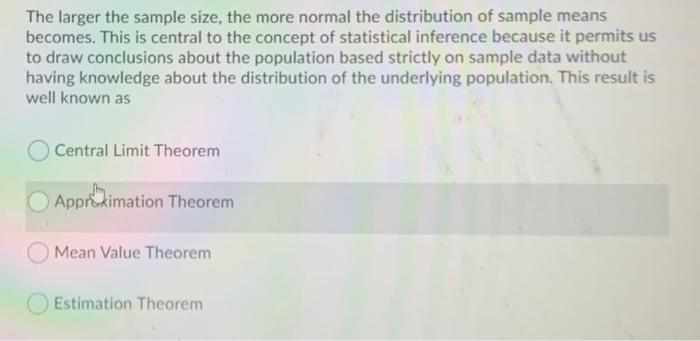# Question The larger the sample size, the more normal the distribution of sample means becomes. This is central to the concept of statistical inference because it permits us to draw conclusions about the population based strictly on sample data without having knowledge about the distribution of the underlying population. This result is well known as Central Limit Theorem Approximation Theorem Mean Value Theorem Estimation TheoremHPGNFP The Asker · Probability and StatisticsTranscribed Image Text: The larger the sample size, the more normal the distribution of sample means becomes. This is central to the concept of statistical inference because it permits us to draw conclusions about the population based strictly on sample data without having knowledge about the distribution of the underlying population. This result is well known as Central Limit Theorem Approximation Theorem Mean Value Theorem Estimation Theorem
More
Transcribed Image Text: The larger the sample size, the more normal the distribution of sample means becomes. This is central to the concept of statistical inference because it permits us to draw conclusions about the population based strictly on sample data without having knowledge about the distribution of the underlying population. This result is well known as Central Limit Theorem Approximation Theorem Mean Value Theorem Estimation Theorem